## 12AX7/12AU7 Hybrid Amplifier circuit test and verification

Below a close picture of the 12AX7 tube powered on.12AX7/12AU7
A picture of the whole board powered on.
Left orange LED is +24V power on, right orange LED is +5V power on.Top sliding switch is +24V on/off, just below is the +5V on/off switch. To the right is the Gain Boost on/off for the power amp 8ohm speaker section, just below, the blue potentiometer, is the output gain control, below is the Gain Boost switch for the headphones/line-out section, below it is the power amp volume, below at the bottom is the headphones/line-out volume.
On the left at the bottom are the three controls for the 3-band equalizer, based on a Fender tone stack: bass, mid and treble. Above the bass control is the input gain (it has no real effect on the 12AX7/12AU7 amplifier.
The 24-pins TSSOP device on the right is the 25W class-D power amplifier.
12AX7 tube is plugged into a socket.12AU7/12AX7 Hybrid 25W Amplifier

### Wave signal verification with oscilloscope

Summary of results:
• First JFET amplifier gain is 0 dB
• First 12AX7 tube stage gain is 10dB
• Second JFET buffer gain is 0 dB
• 3-band equalizer gain at 1kHz with Bass, Mid and Top = 0.5 is -17dB
• Second 12AX7 tube stage gain is 8.5 dB
• Third JFET amplifier gain with gain control and Boost Off:
• G2=0.0 =>-22 dB
• G2=0.5 => -8 dB
• G2=1.0 => -5 dB
• Third JFET amplifier gain with gain control and Boost ON:
• G2=0.0 => 3 dB
• G2=0.5 => 17 dB
• G2=1.0 => 20 dB
Maximum output level is 7880mVpp which corresponds to a maximum total gain of 22.4dB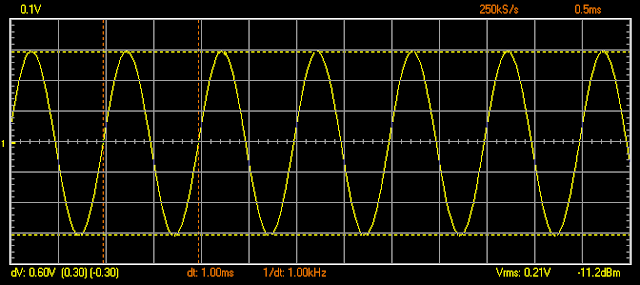Input signal: Sine wave 600mVpp 1kHzTP2 signal after first JFET buffer 600 mVpp  (0 dB)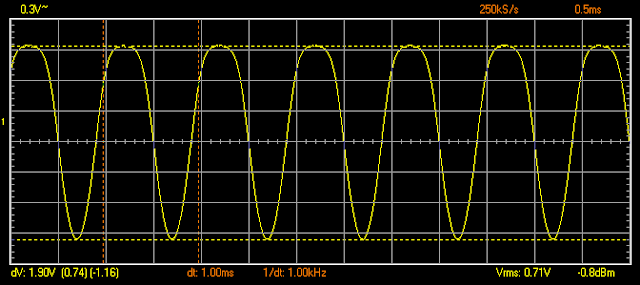TP4 signal after first triode anode 1900mVpp  (10 dB)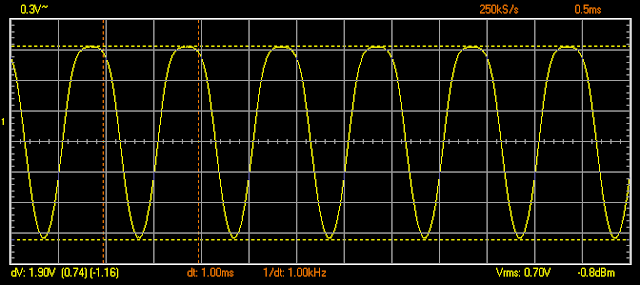C16 signal after second JFET buffer 1900mVpp (0 dB)C20 signal after 3-band equalizer 280mVpp. Treble=Middle=Bass = 0.5  (-17 dB)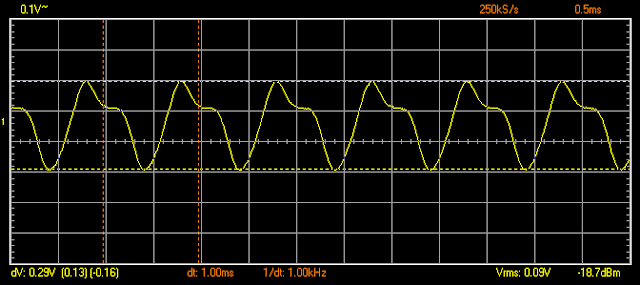TP21 signal after third JFET buffer 290mVpp (0 dB)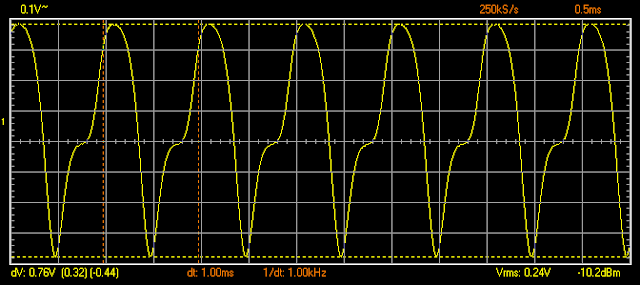TP5 signal after second triode anode 760mVpp (8.5 dB)C27 signal after fourth JFET amplifier 57mV, Gain 2 = 0 (-22.5 dB)C27 signal after fourth JFET amplifier 300mV, Gain 2 = 0.5 (-8 dB)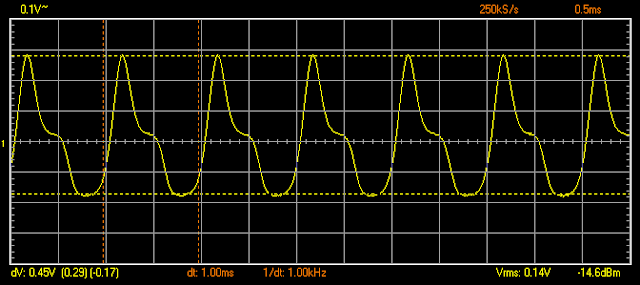C27 signal after fourth JFET amplifier 450mV, Gain 2 = 1.0 (-4.6 dB)C27 signal after fourth JFET amplifier 1100mV, Gain 2 = 0.0. BOOST ON (3.2 dB)C27 signal after fourth JFET amplifier 5630mV, Gain 2 = 0.5. BOOST ON (17.4 dB)C27 signal after fourth JFET amplifier 7880mV, Gain 2 = 1.0. BOOST ON (20.3 dB)